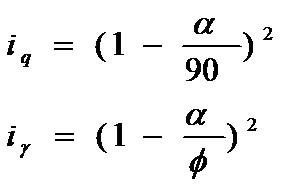# HOW TO CALCULATE BEARING CAPACITY OF SHALLOW FOUNDATION IN SAND FROM SPT VALUES OR N-VALUES (IS-6403)

## Step-by-Step Procedure for Calculation of Bearing Capacity from Standard Penetration Test Values or N-values

### Step-1

Perform standard penetration test on the location for which you want to calculate bearing capacity. This is done as per standard procedure given in IS-2131. Standard penetration test must be done at every 75 cm in vertical direction.

### Step-2

Decide the depth, width and length of foundation for initial calculation. This is a trial and error process. In the first attempt, you can never get the exact size of foundation which will satisfy all of your needs.

### Step-3

Apply necessary corrections to the standard penetration test values. Calculate the cumulative average value of corrected SPT values from the base level of foundation to a depth equal to 2 times the width of foundation.

### Step-4

Correlate the above cumulative average SPT value with the fig given below to find out the corresponding angle of shearing resistance (ϕ).

### Step-5

Calculate effective surcharge at the base level of foundation by multiplying the effective unit weight of soil with the depth of the foundation i.e.

q = ϒ*Df

Where,

q = Effective surcharge at the base level of foundation, in kgf/cm2

ϒ = Unit weight of soil, in kgf/cm3

Df = Depth of foundation, in cm

### Step-6

For the angle of shearing resistance value (ϕ) as calculated in step-4, find out the corresponding values of bearing capacity factors (i.e. Nq & Nϒ) from the table given below. For the intermediate values of ‘ϕ’, make linear interpolation.

### Step-7

Calculate shape factors (i.e. sq & sϒ) using formula given below.Where,

B = Width of foundation, in cm

L = Length of foundation, in cm

### Step-8

Calculate depth factors (i.e. dq & dϒ) using following formula.

dq=dϒ=1 (for ϕ < 100)

dq = dϒ = 1+0.1(Df/B)(Nϕ)1/2 (for ϕ>100)

Nϕ is calculated using following formula

Nϕ = tan2[(π/4)+(ϕ/2)]

### Step-9

Calculate inclination factors (i.e. iq & iϒ) using the formula given belowWhere,

α = Inclination of the load to the vertical in degrees

ϕ = Angles of shearing resistance in degrees

### Step-10

Calculate the correction factor for location of water table using the following formula

W’ = 0.5+0.5[Dw/(Df+B)]

Where,

W’ = Correction factor for location of water table

Dw = Depth of water table, in cm

Df = Depth of foundation, in cm

### Step-11

Using the equation given below calculate the net ultimate bearing capacity.Where,

qd = Net ultimate bearing capacity of foundation, kgf/cm2

q = Effective surcharge at base level of foundation, in kgf/cm2 (Refer step-5)

Nq & Nϒ = Bearing capacity factors (Refer step-6)

sq & sϒ = Shape factors (Refer step-7)

dq & dϒ = Depth factors (Refer step-8)

iq & iϒ = Inclination factors (Refer step-9)

W’ = Correction factor for location of water table (Refer step-10)

B = Width of foundation, in cm

ϒ = Bulk unit weight of foundation soil, in kgf/cm3.

1.2.3.4.5.6.7.8.•9.10.11.12.